# Digital Logic Design I Chapter 1 Digital Systems

• Slides: 55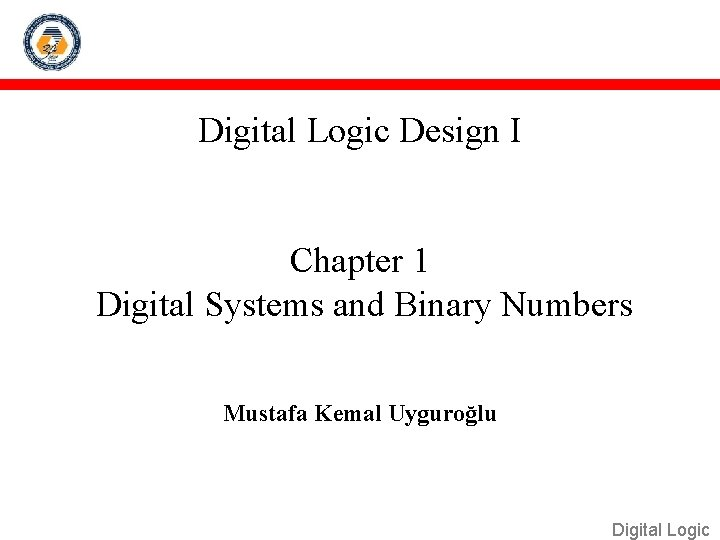Digital Logic Design I Chapter 1 Digital Systems and Binary Numbers Mustafa Kemal Uyguroğlu Digital Logic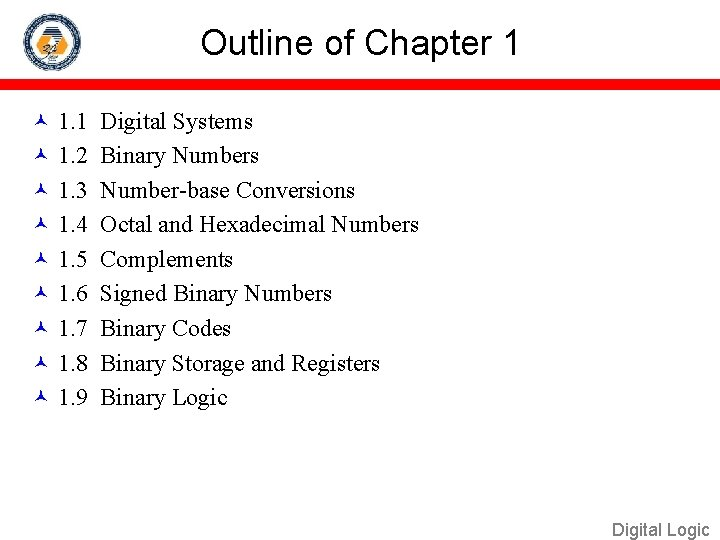Outline of Chapter 1 1. 1 Digital Systems 1. 2 Binary Numbers 1. 3 Number-base Conversions 1. 4 Octal and Hexadecimal Numbers 1. 5 Complements 1. 6 Signed Binary Numbers 1. 7 Binary Codes 1. 8 Binary Storage and Registers 1. 9 Binary Logic Digital Logic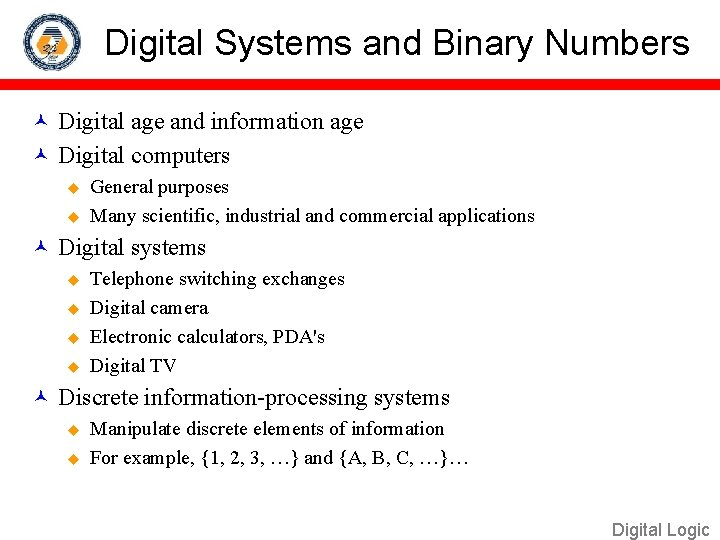Digital Systems and Binary Numbers Digital age and information age Digital computers u u General purposes Many scientific, industrial and commercial applications Digital systems u u Telephone switching exchanges Digital camera Electronic calculators, PDA's Digital TV Discrete information-processing systems u u Manipulate discrete elements of information For example, {1, 2, 3, …} and {A, B, C, …}… Digital LogicAnalog and Digital Signal Analog system u The physical quantities or signals may vary continuously over a specified range. Digital system u u The physical quantities or signals can assume only discrete values. Greater accuracy X(t) t Analog signal t Digital signal Digital LogicBinary Digital Signal An information variable represented by physical quantity. For digital systems, the variable takes on discrete values. u Two level, or binary values are the most prevalent values. Binary values are represented abstractly by: u u Digits 0 and 1 Words (symbols) False (F) and True (T) Words (symbols) Low (L) and High (H) And words On and Off V(t) Logic 1 Binary values are represented by values or ranges of values of physical quantities. undefine Logic 0 t Binary digital signal Digital LogicDecimal Number System Base (also called radix) = 10 u 10 digits { 0, 1, 2, 3, 4, 5, 6, 7, 8, 9 } Digit Position u Integer & fraction Digit Weight u Weight = (Base) Position Magnitude u 2 1 0 5 1 2 100 -1 -2 7 4 10 1 0. 01 10 2 0. 7 0. 04 Sum of “Digit x Weight” Formal Notation 500 d 2*B 2+d 1*B 1+d 0*B 0+d-1*B-1+d-2*B-2 (512. 74)10 Digital Logic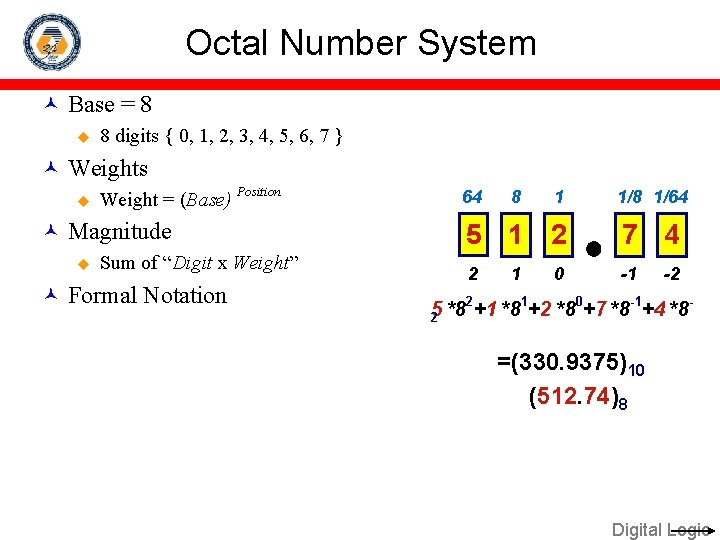Octal Number System Base = 8 u 8 digits { 0, 1, 2, 3, 4, 5, 6, 7 } Weights u Weight = (Base) Position Magnitude u Sum of “Digit x Weight” Formal Notation 64 8 1 1/8 1/64 5 1 2 7 4 2 -1 1 0 -2 2 1 0 -1 5 *8 +1 *8 +2 *8 +7 *8 +4 *8 2 =(330. 9375)10 (512. 74)8 Digital Logic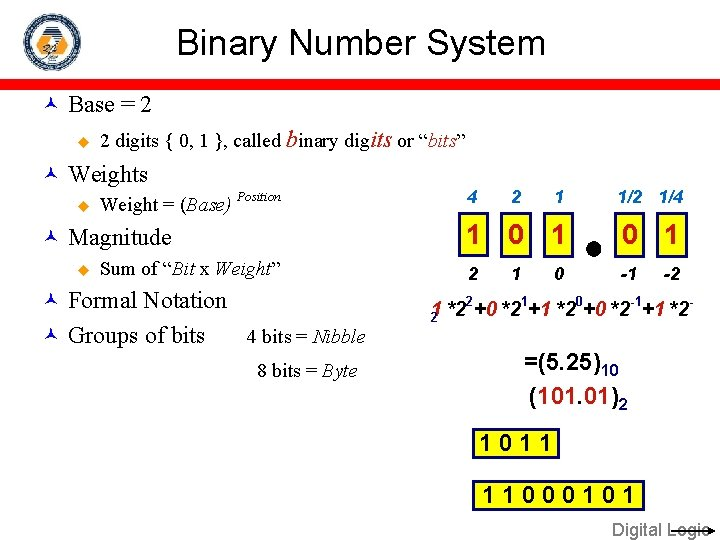Binary Number System Base = 2 u 2 digits { 0, 1 }, called binary digits or “bits” Weights u Weight = (Base) Position Magnitude u Sum of “Bit x Weight” Formal Notation Groups of bits 4 bits = Nibble 8 bits = Byte 4 2 1 1/2 1/4 1 0 1 2 -1 1 0 -2 2 1 0 -1 1 *2 +0 *2 +1 *2 2 =(5. 25)10 (101. 01)2 1011 11000101 Digital LogicHexadecimal Number System Base = 16 u 16 digits { 0, 1, 2, 3, 4, 5, 6, 7, 8, 9, A, B, C, D, E, F } Weights u Weight = (Base) Position Magnitude u Sum of “Digit x Weight” Formal Notation 256 16 1 1/16 1/256 1 E 5 7 A 2 -1 1 0 -2 1 *162+14 *161+5 *160+7 *16 -1+10 *16 -2 =(485. 4765625)10 (1 E 5. 7 A)16 Digital Logic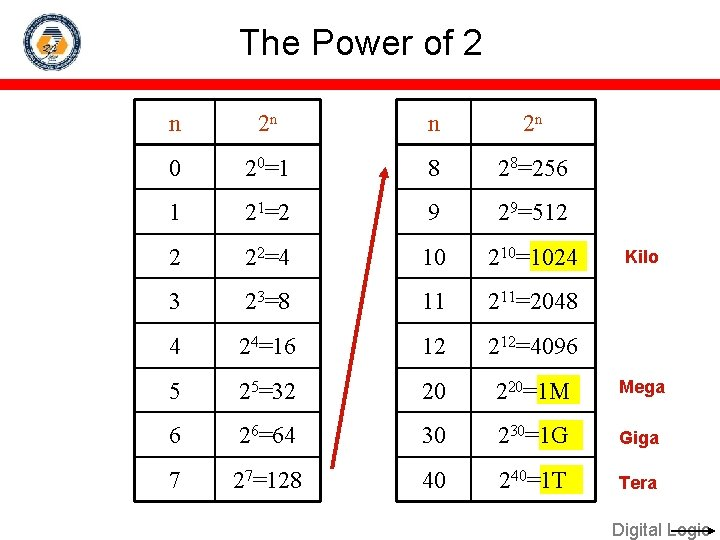The Power of 2 n 2 n 0 20=1 8 28=256 1 21=2 9 29=512 2 22=4 10 210=1024 3 23=8 11 211=2048 4 24=16 12 212=4096 5 25=32 20 220=1 M Mega 6 26=64 30 230=1 G Giga 7 27=128 40 240=1 T Tera Kilo Digital LogicAddition Decimal Addition 1 + 1 1 Carry 5 5 1 0 = Ten ≥ Base Subtract a Base Digital LogicBinary Addition Column Addition 1 1 1 1 1 0 1 = 61 1 0 1 1 1 = 23 1 0 0 = 84 + 1 0 ≥ (2)10 Digital LogicBinary Subtraction Borrow a “Base” when needed 0 1 2 2 0 0 2 1 0 0 1 1 0 1 = 77 1 0 1 1 1 = 23 1 0 1 1 0 = 54 − 0 1 2 = (10)2 Digital Logic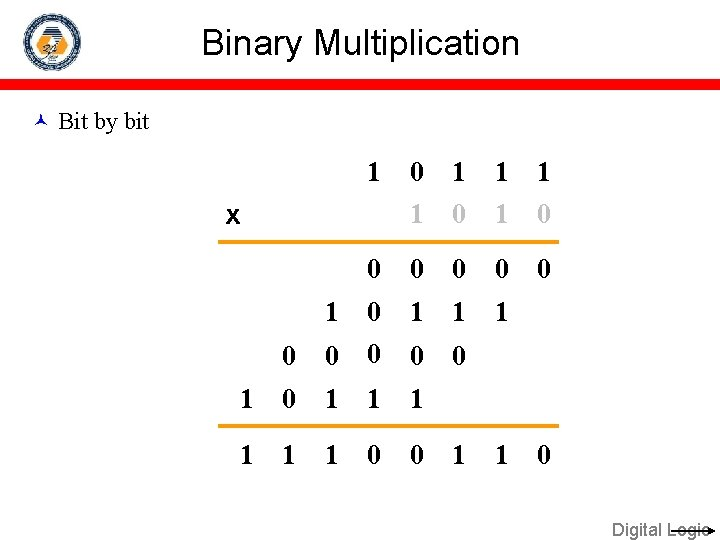Binary Multiplication Bit by bit 1 0 1 0 0 0 1 0 1 1 1 0 0 0 1 1 1 1 0 0 x 1 1 0 Digital LogicNumber Base Conversions Evaluate Magnitude Octal (Base 8) Evaluate Magnitude Decimal (Base 10) Binary (Base 2) Hexadecimal (Base 16) Evaluate Magnitude Digital LogicDecimal (Integer) to Binary Conversion Divide the number by the ‘Base’ (=2) Take the remainder (either 0 or 1) as a coefficient Take the quotient and repeat the division Example: (13)10 Quotient Remainder Coefficient 6 3 1 0 1 1 a 0 = 1 a 1 = 0 a 2 = 1 a 3 = 1 13/ 2 = 6 /2= 3 /2= 1 /2= Answer: (13)10 = (a 3 a 2 a 1 a 0)2 = (1101)2 MSB LSB Digital Logic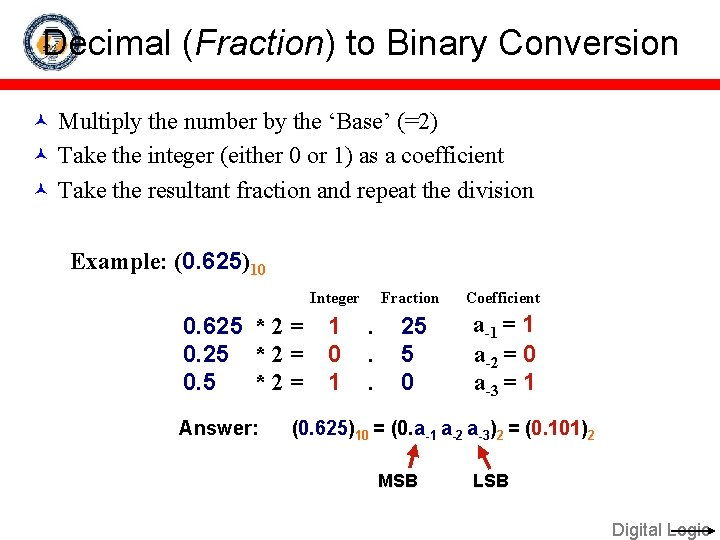Decimal (Fraction) to Binary Conversion Multiply the number by the ‘Base’ (=2) Take the integer (either 0 or 1) as a coefficient Take the resultant fraction and repeat the division Example: (0. 625)10 Integer 0. 625 * 2 = 0. 5 *2= Answer: 1 0 1 . . . Fraction Coefficient 25 5 0 a-1 = 1 a-2 = 0 a-3 = 1 (0. 625)10 = (0. a-1 a-2 a-3)2 = (0. 101)2 MSB LSB Digital LogicDecimal to Octal Conversion Example: (175)10 Quotient 175 / 8 = 21 / 8 = 2 /8= Remainder Coefficient 7 5 2 a 0 = 7 a 1 = 5 a 2 = 2 21 2 0 Answer: (175)10 = (a 2 a 1 a 0)8 = (257)8 Example: (0. 3125)10 Integer 0. 3125 * 8 = 2 0. 5 *8= 4 Answer: . . Fraction Coefficient 5 0 a-1 = 2 a-2 = 4 (0. 3125)10 = (0. a-1 a-2 a-3)8 = (0. 24)8 Digital Logic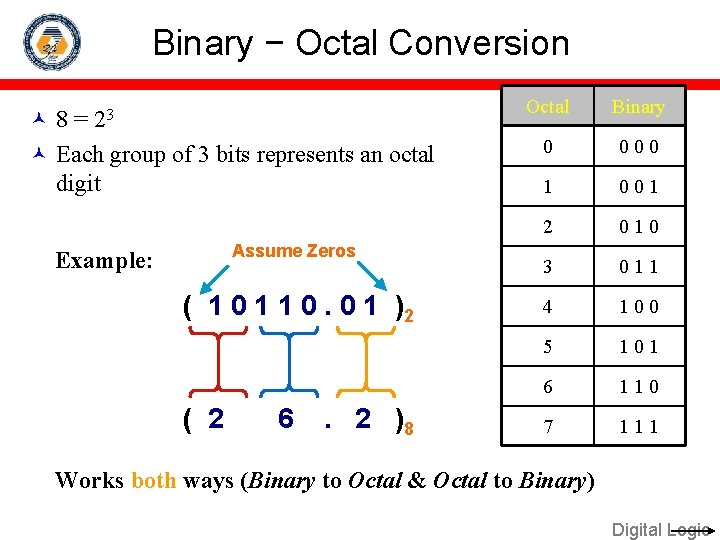Binary − Octal Conversion 8 = 23 Each group of 3 bits represents an octal digit Assume Zeros Example: ( 1 0 1 1 0. 0 1 )2 ( 2 6 . 2 )8 Octal Binary 0 0 1 2 0 1 0 3 0 1 1 4 1 0 0 5 1 0 1 6 1 1 0 7 1 1 1 Works both ways (Binary to Octal & Octal to Binary) Digital Logic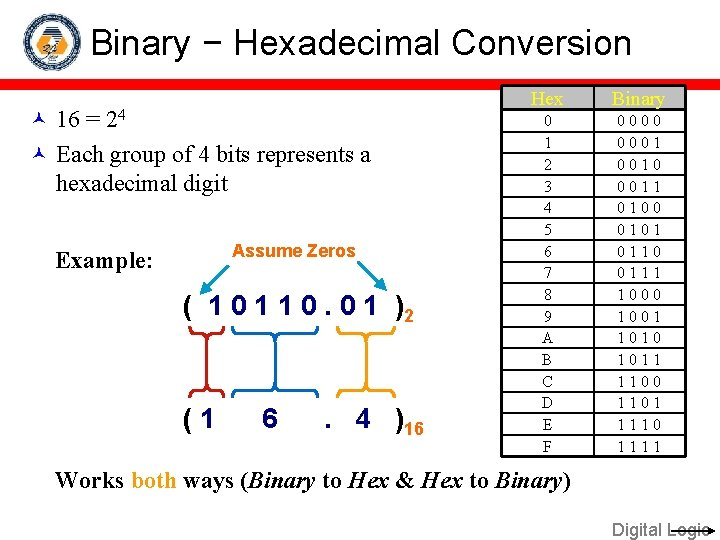Binary − Hexadecimal Conversion 16 = 24 Each group of 4 bits represents a hexadecimal digit Assume Zeros Example: ( 1 0 1 1 0. 0 1 )2 (1 6 . 4 )16 Hex Binary 0 1 2 3 4 5 6 7 8 9 A B C D E F 0 0 0 0 1 1 0 0 0 1 0 1 1 0 0 0 1 1 0 1 0 1 1 0 0 1 1 1 1 0 1 1 Works both ways (Binary to Hex & Hex to Binary) Digital LogicOctal − Hexadecimal Conversion Convert to Binary as an intermediate step Example: ( 2 6 . 2 )8 Assume Zeros ( 0 1 1 0. 0 1 0 )2 (1 6 . 4 )16 Works both ways (Octal to Hex & Hex to Octal) Digital Logic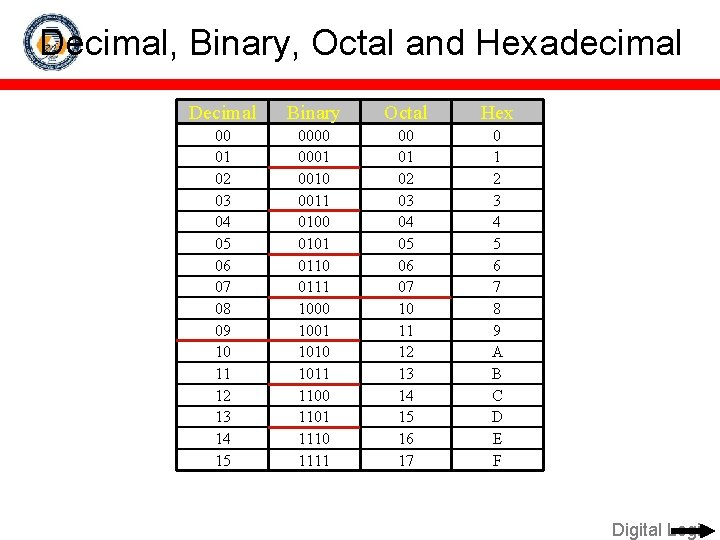Decimal, Binary, Octal and Hexadecimal Decimal Binary Octal Hex 00 01 02 03 04 05 06 07 08 09 10 11 12 13 14 15 0000 0001 0010 0011 0100 0101 0110 0111 1000 1001 1010 1011 1100 1101 1110 1111 00 01 02 03 04 05 06 07 10 11 12 13 14 15 16 17 0 1 2 3 4 5 6 7 8 9 A B C D E F Digital Logic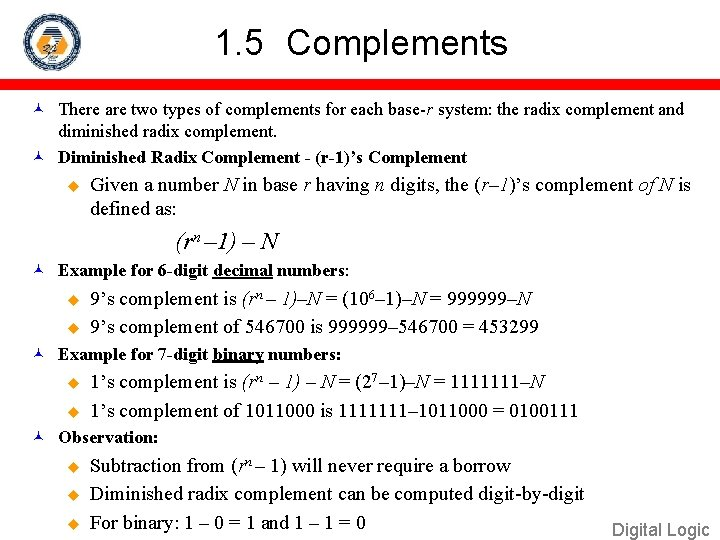1. 5 Complements There are two types of complements for each base-r system: the radix complement and diminished radix complement. Diminished Radix Complement - (r-1)’s Complement u Given a number N in base r having n digits, the (r– 1)’s complement of N is defined as: (rn – 1) – N Example for 6 -digit decimal numbers: u u 9’s complement is (rn – 1)–N = (106– 1)–N = 999999–N 9’s complement of 546700 is 999999– 546700 = 453299 Example for 7 -digit binary numbers: u u 1’s complement is (rn – 1) – N = (27– 1)–N = 1111111–N 1’s complement of 1011000 is 1111111– 1011000 = 0100111 Observation: u u u Subtraction from (rn – 1) will never require a borrow Diminished radix complement can be computed digit-by-digit For binary: 1 – 0 = 1 and 1 – 1 = 0 Digital LogicComplements 1’s Complement (Diminished Radix Complement) All ‘ 0’s become ‘ 1’s u All ‘ 1’s become ‘ 0’s Example (10110000)2 (01001111)2 If you add a number and its 1’s complement … u 10110000 + 01001111 Digital Logic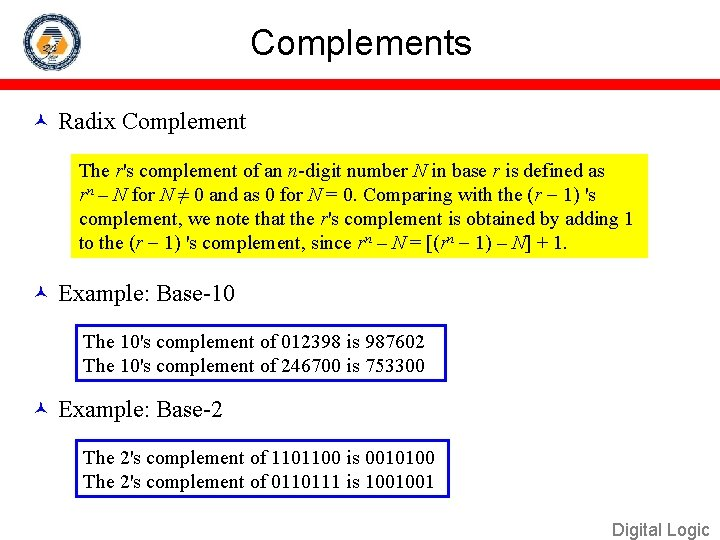Complements Radix Complement The r's complement of an n-digit number N in base r is defined as rn – N for N ≠ 0 and as 0 for N = 0. Comparing with the (r 1) 's complement, we note that the r's complement is obtained by adding 1 to the (r 1) 's complement, since rn – N = [(rn 1) – N] + 1. Example: Base-10 The 10's complement of 012398 is 987602 The 10's complement of 246700 is 753300 Example: Base-2 The 2's complement of 1101100 is 0010100 The 2's complement of 0110111 is 1001001 Digital Logic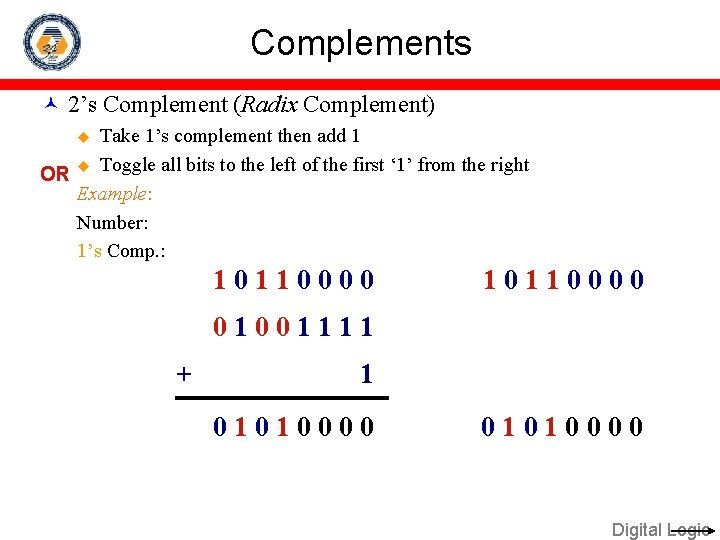Complements 2’s Complement (Radix Complement) Take 1’s complement then add 1 OR u Toggle all bits to the left of the first ‘ 1’ from the right Example: Number: 1’s Comp. : u 10110000 01001111 + 1 01010000 Digital LogicComplements Subtraction with Complements u The subtraction of two n-digit unsigned numbers M – N in base r can be done as follows: Digital Logic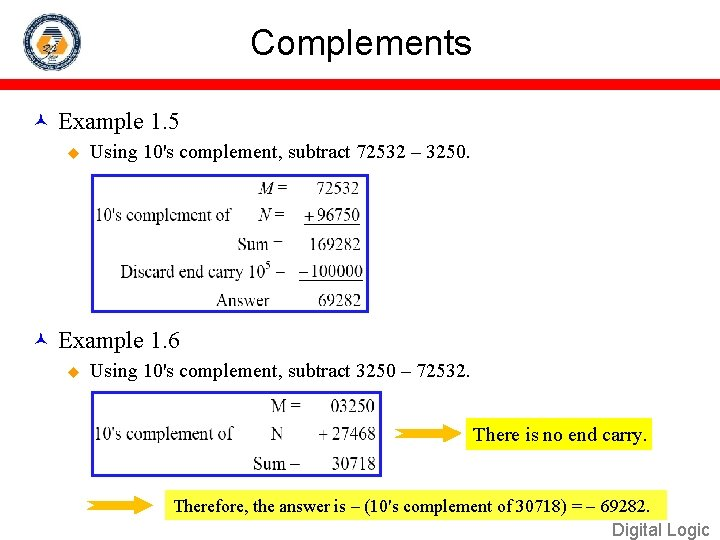Complements Example 1. 5 u Using 10's complement, subtract 72532 – 3250. Example 1. 6 u Using 10's complement, subtract 3250 – 72532. There is no end carry. Therefore, the answer is – (10's complement of 30718) = 69282. Digital Logic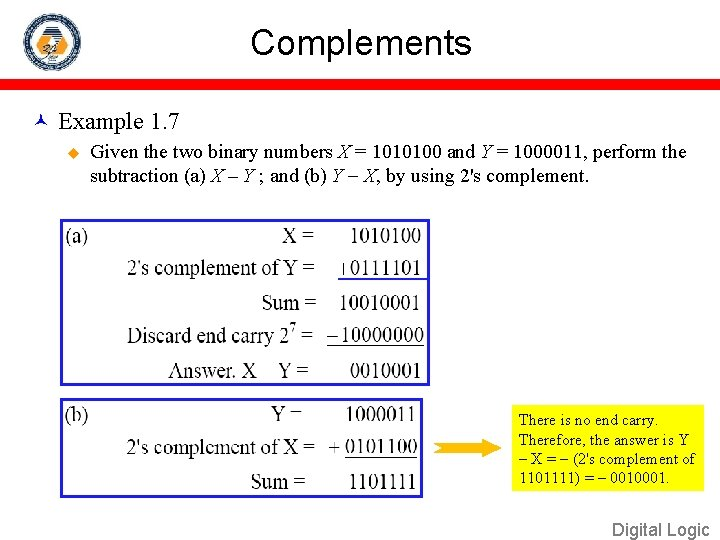Complements Example 1. 7 u Given the two binary numbers X = 1010100 and Y = 1000011, perform the subtraction (a) X – Y ; and (b) Y X, by using 2's complement. There is no end carry. Therefore, the answer is Y – X = (2's complement of 1101111) = 0010001. Digital Logic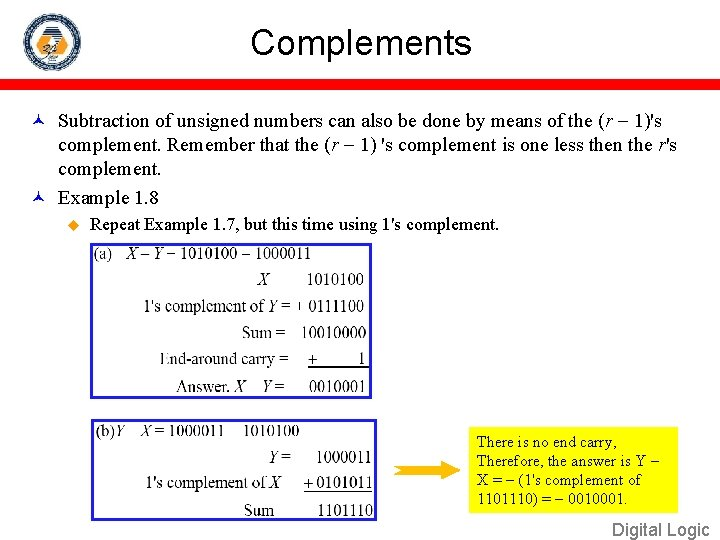Complements Subtraction of unsigned numbers can also be done by means of the (r 1)'s complement. Remember that the (r 1) 's complement is one less then the r's complement. Example 1. 8 u Repeat Example 1. 7, but this time using 1's complement. There is no end carry, Therefore, the answer is Y – X = (1's complement of 1101110) = 0010001. Digital Logic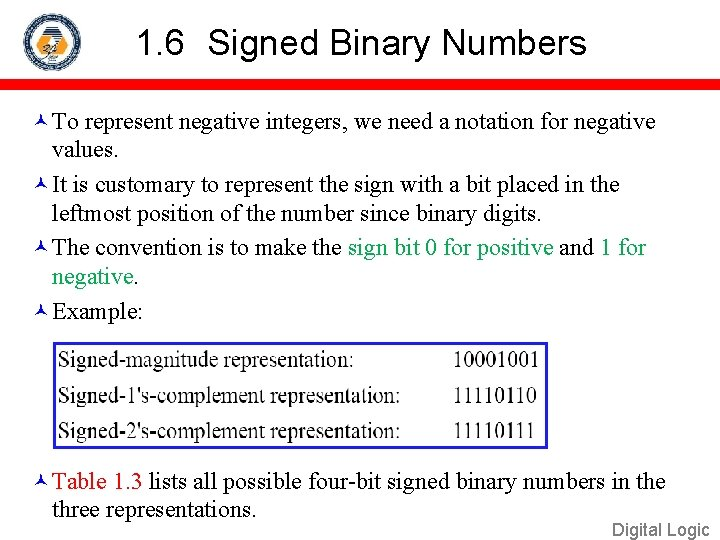1. 6 Signed Binary Numbers To represent negative integers, we need a notation for negative values. It is customary to represent the sign with a bit placed in the leftmost position of the number since binary digits. The convention is to make the sign bit 0 for positive and 1 for negative. Example: Table 1. 3 lists all possible four-bit signed binary numbers in the three representations. Digital LogicSigned Binary Numbers Digital LogicSigned Binary Numbers Arithmetic addition u The addition of two numbers in the signed-magnitude system follows the rules of ordinary arithmetic. If the signs are the same, we add the two magnitudes and give the sum the common sign. If the signs are different, we subtract the smaller magnitude from the larger and give the difference the sign if the larger magnitude. u The addition of two signed binary numbers with negative numbers represented in signed-2's-complement form is obtained from the addition of the two numbers, including their sign bits. u A carry out of the sign-bit position is discarded. Example: Digital Logic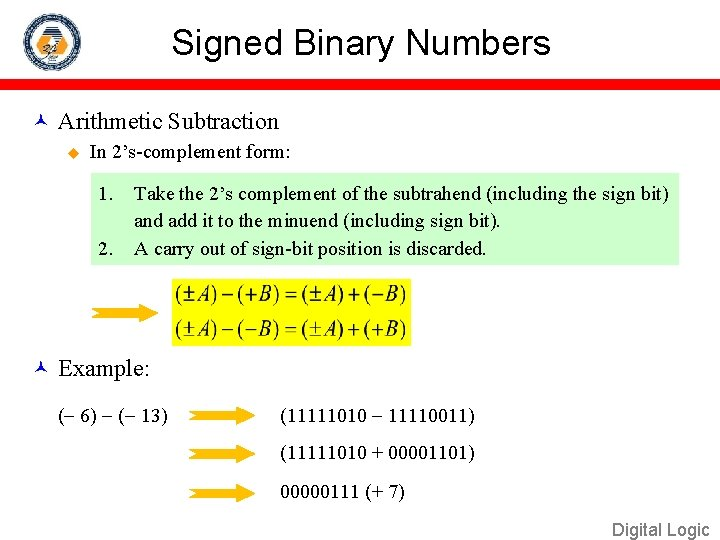Signed Binary Numbers Arithmetic Subtraction u In 2’s-complement form: 1. 2. Take the 2’s complement of the subtrahend (including the sign bit) and add it to the minuend (including sign bit). A carry out of sign-bit position is discarded. Example: ( 6) ( 13) (11111010 11110011) (11111010 + 00001101) 00000111 (+ 7) Digital Logic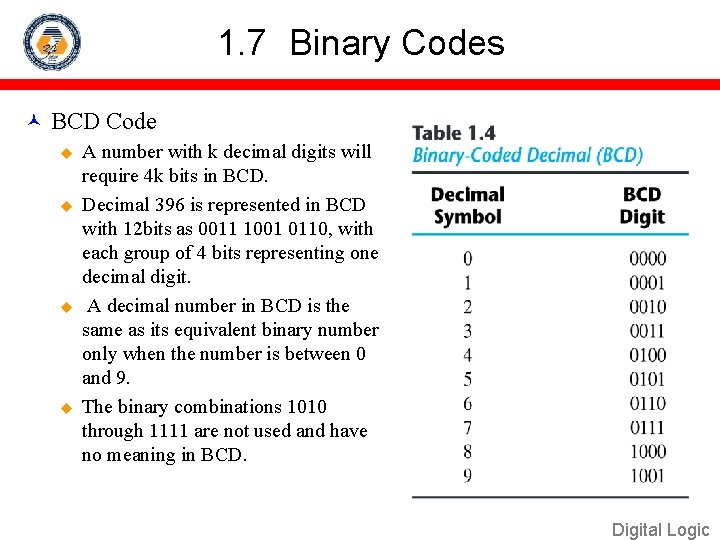1. 7 Binary Codes BCD Code u u A number with k decimal digits will require 4 k bits in BCD. Decimal 396 is represented in BCD with 12 bits as 0011 1001 0110, with each group of 4 bits representing one decimal digit. A decimal number in BCD is the same as its equivalent binary number only when the number is between 0 and 9. The binary combinations 1010 through 1111 are not used and have no meaning in BCD. Digital Logic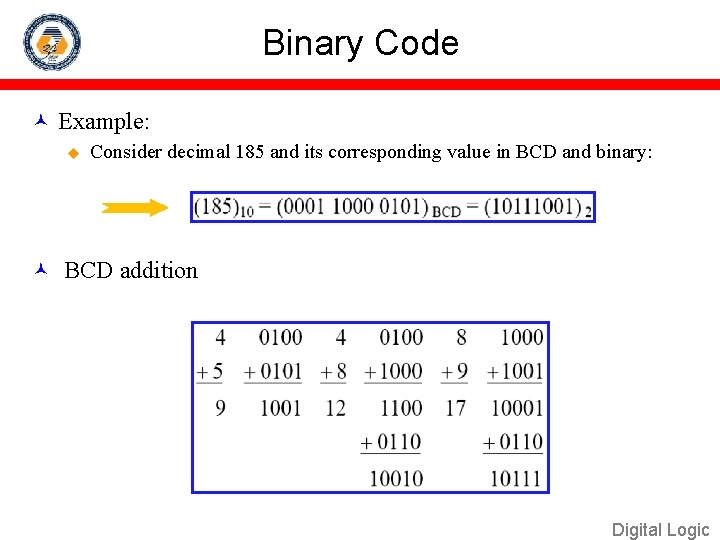Binary Code Example: u Consider decimal 185 and its corresponding value in BCD and binary: BCD addition Digital Logic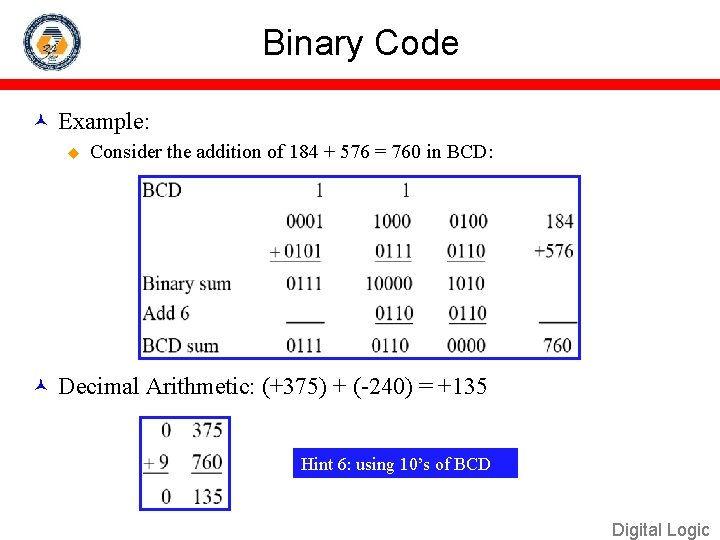Binary Code Example: u Consider the addition of 184 + 576 = 760 in BCD: Decimal Arithmetic: (+375) + (-240) = +135 Hint 6: using 10’s of BCD Digital LogicBinary Codes Other Decimal Codes Digital Logic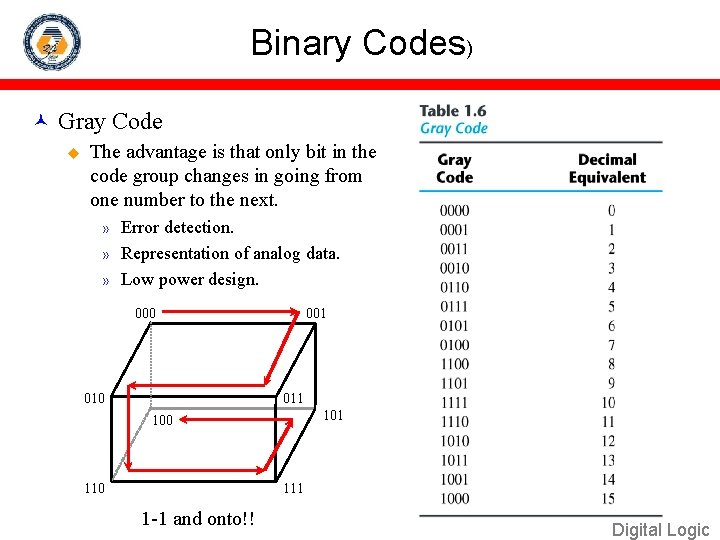Binary Codes) Gray Code u The advantage is that only bit in the code group changes in going from one number to the next. Error detection. » Representation of analog data. » Low power design. » 000 010 001 011 100 111 1 -1 and onto!! Digital Logic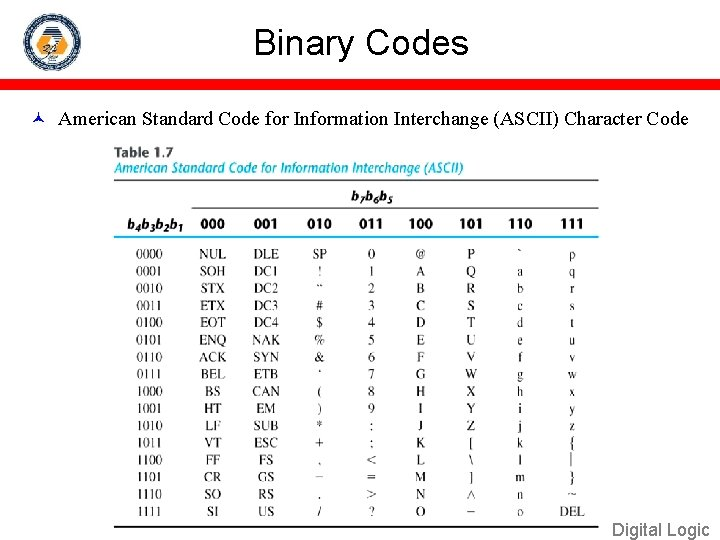Binary Codes American Standard Code for Information Interchange (ASCII) Character Code Digital LogicBinary Codes ASCII Character Code Digital LogicASCII Character Codes American Standard Code for Information Interchange (Refer to Table 1. 7) A popular code used to represent information sent as characterbased data. It uses 7 -bits to represent: u u 94 Graphic printing characters. 34 Non-printing characters. Some non-printing characters are used for text format (e. g. BS = Backspace, CR = carriage return). Other non-printing characters are used for record marking and flow control (e. g. STX and ETX start and end text areas). Digital Logic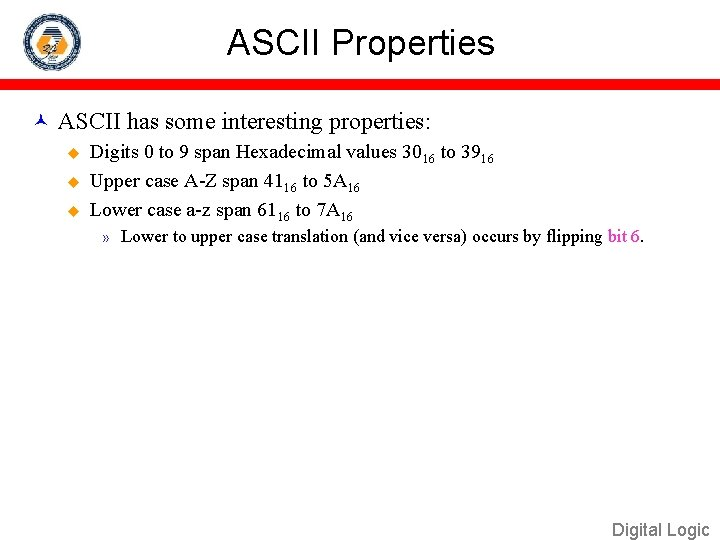ASCII Properties ASCII has some interesting properties: u u u Digits 0 to 9 span Hexadecimal values 3016 to 3916 Upper case A-Z span 4116 to 5 A 16 Lower case a-z span 6116 to 7 A 16 » Lower to upper case translation (and vice versa) occurs by flipping bit 6. Digital LogicBinary Codes Error-Detecting Code u u To detect errors in data communication and processing, an eighth bit is sometimes added to the ASCII character to indicate its parity. A parity bit is an extra bit included with a message to make the total number of 1's either even or odd. Example: u Consider the following two characters and their even and odd parity: Digital Logic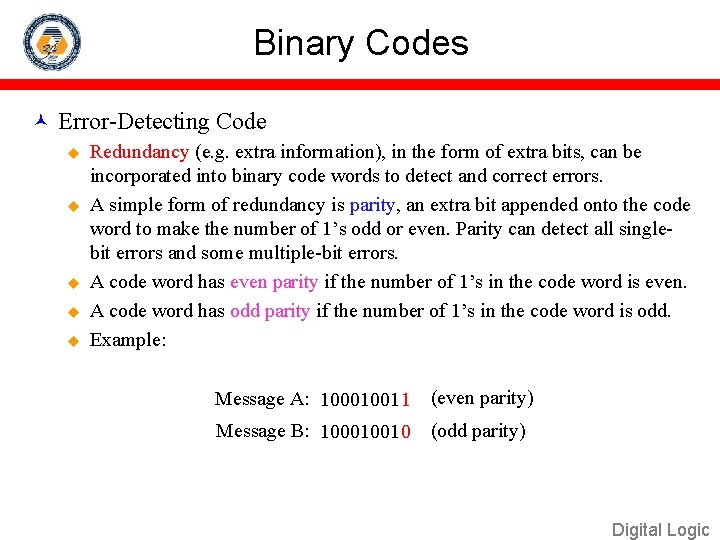Binary Codes Error-Detecting Code u u u Redundancy (e. g. extra information), in the form of extra bits, can be incorporated into binary code words to detect and correct errors. A simple form of redundancy is parity, an extra bit appended onto the code word to make the number of 1’s odd or even. Parity can detect all singlebit errors and some multiple-bit errors. A code word has even parity if the number of 1’s in the code word is even. A code word has odd parity if the number of 1’s in the code word is odd. Example: Message A: 100010011 (even parity) Message B: 10001001 0 (odd parity) Digital Logic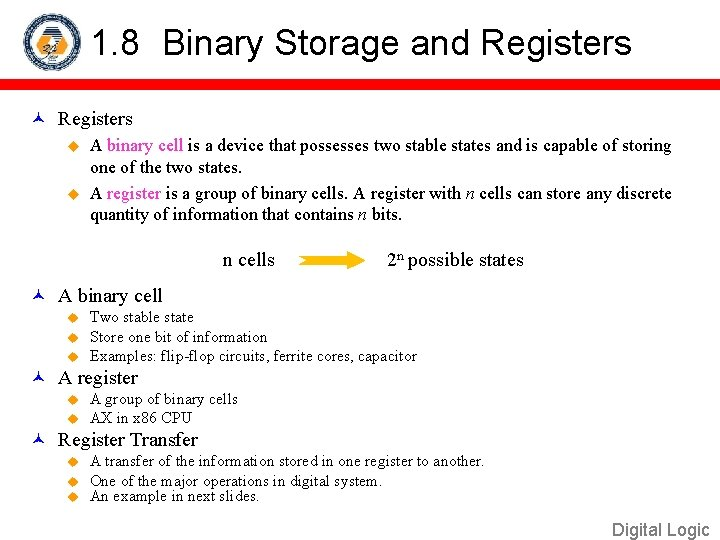1. 8 Binary Storage and Registers u A binary cell is a device that possesses two stable states and is capable of storing one of the two states. u A register is a group of binary cells. A register with n cells can store any discrete quantity of information that contains n bits. n cells 2 n possible states A binary cell u u u Two stable state Store one bit of information Examples: flip-flop circuits, ferrite cores, capacitor A register u u A group of binary cells AX in x 86 CPU Register Transfer u u u A transfer of the information stored in one register to another. One of the major operations in digital system. An example in next slides. Digital LogicA Digital Computer Example Outputs: CRT, LCD, modem, speakers Inputs: Keyboard, mouse, modem, microphone Synchronous or Asynchronous? Digital Logic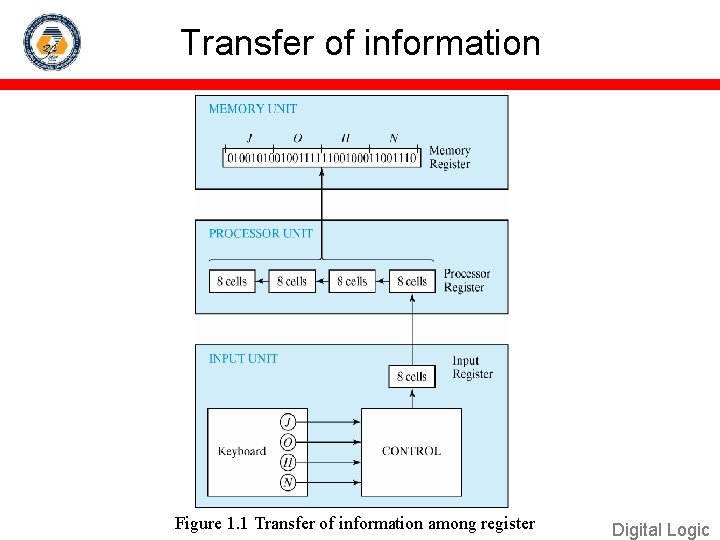Transfer of information Figure 1. 1 Transfer of information among register Digital Logic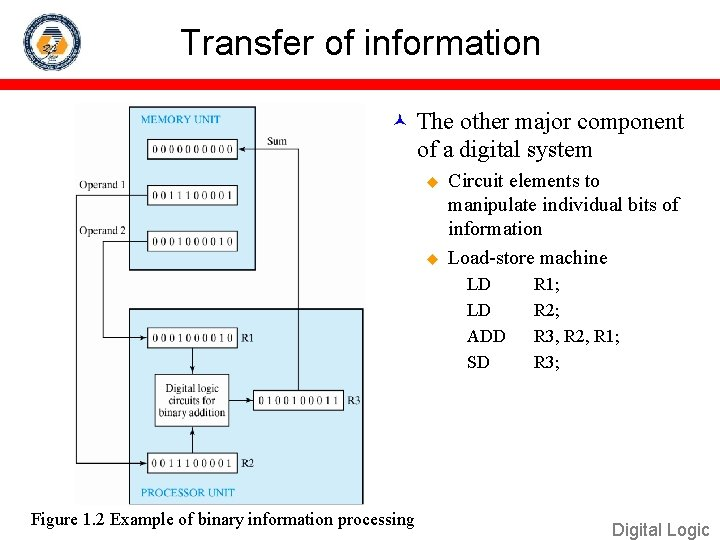Transfer of information The other major component of a digital system u u Circuit elements to manipulate individual bits of information Load-store machine LD LD ADD SD Figure 1. 2 Example of binary information processing R 1; R 2; R 3, R 2, R 1; R 3; Digital Logic1. 9 Binary Logic Definition of Binary Logic u Binary logic consists of binary variables and a set of logical operations. u The variables are designated by letters of the alphabet, such as A, B, C, x, y, z, etc, with each variable having two and only two distinct possible values: 1 and 0, u Three basic logical operations: AND, OR, and NOT. Digital LogicBinary Logic Truth Tables, Boolean Expressions, and Logic Gates AND OR NOT x y z x z 0 0 0 0 1 1 1 0 0 1 1 1 1 z=x • y=xy z=x+y z = x’ Digital Logic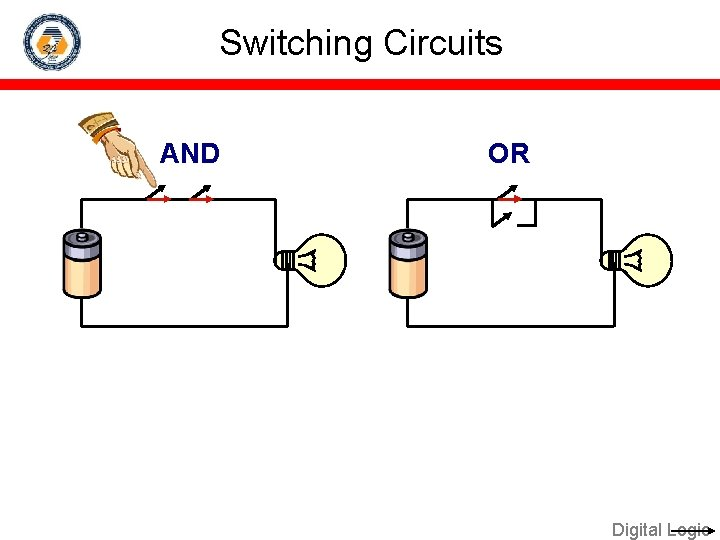Switching Circuits AND OR Digital LogicBinary Logic gates u Example of binary signals 3 Logic 1 2 Un-define 1 0 Logic 0 Figure 1. 3 Example of binary signals Digital LogicBinary Logic gates u Graphic Symbols and Input-Output Signals for Logic gates: Fig. 1. 4 Symbols for digital logic circuits Fig. 1. 5 Input-Output signals for gates Digital Logic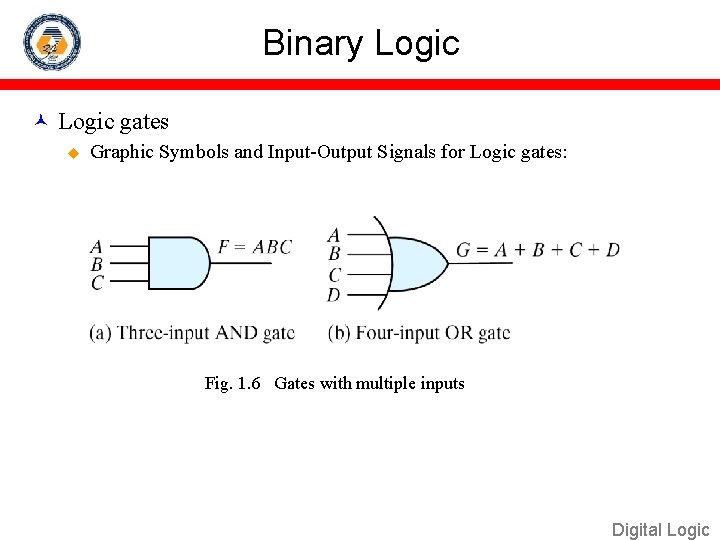Binary Logic gates u Graphic Symbols and Input-Output Signals for Logic gates: Fig. 1. 6 Gates with multiple inputs Digital Logic